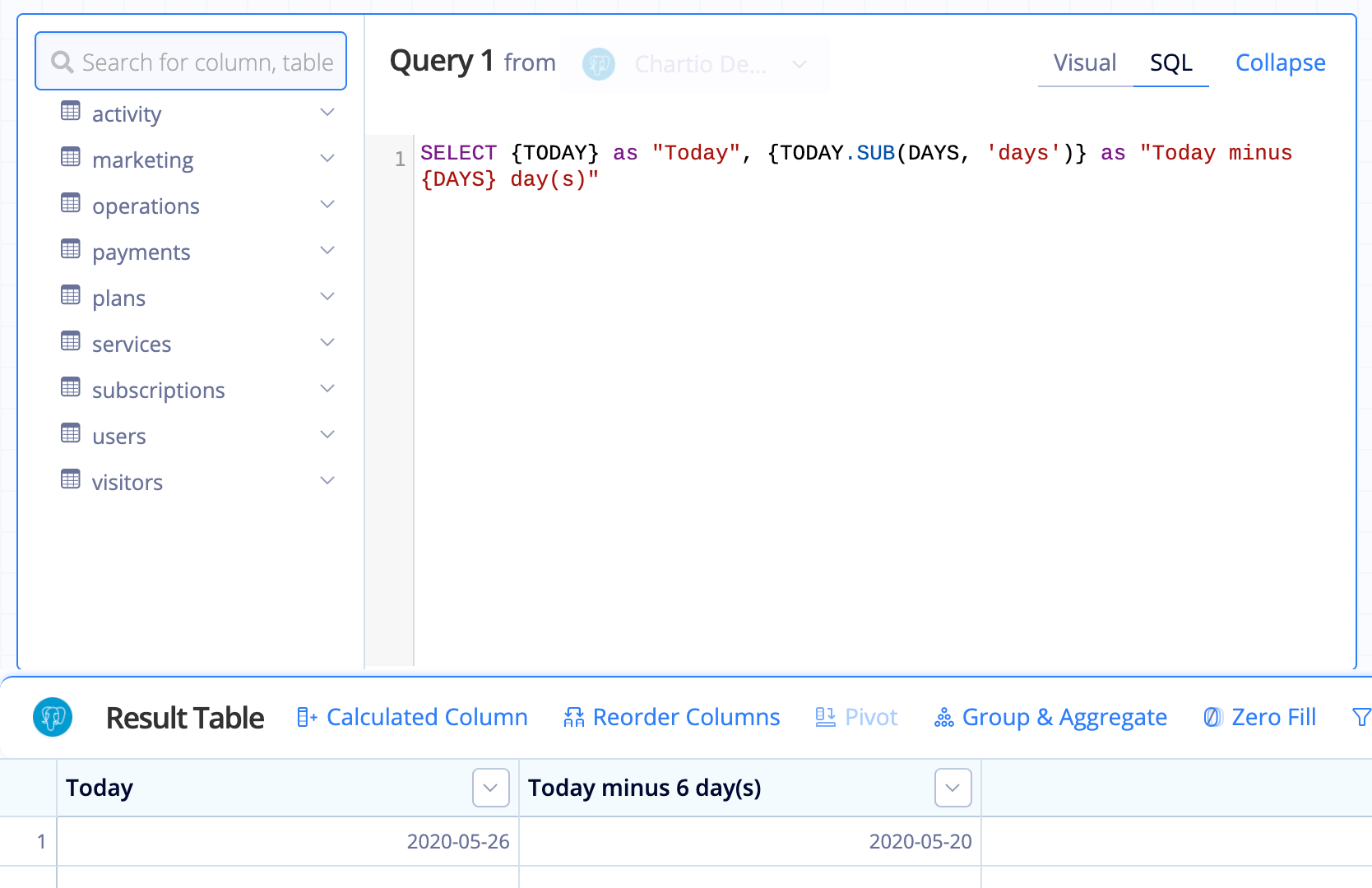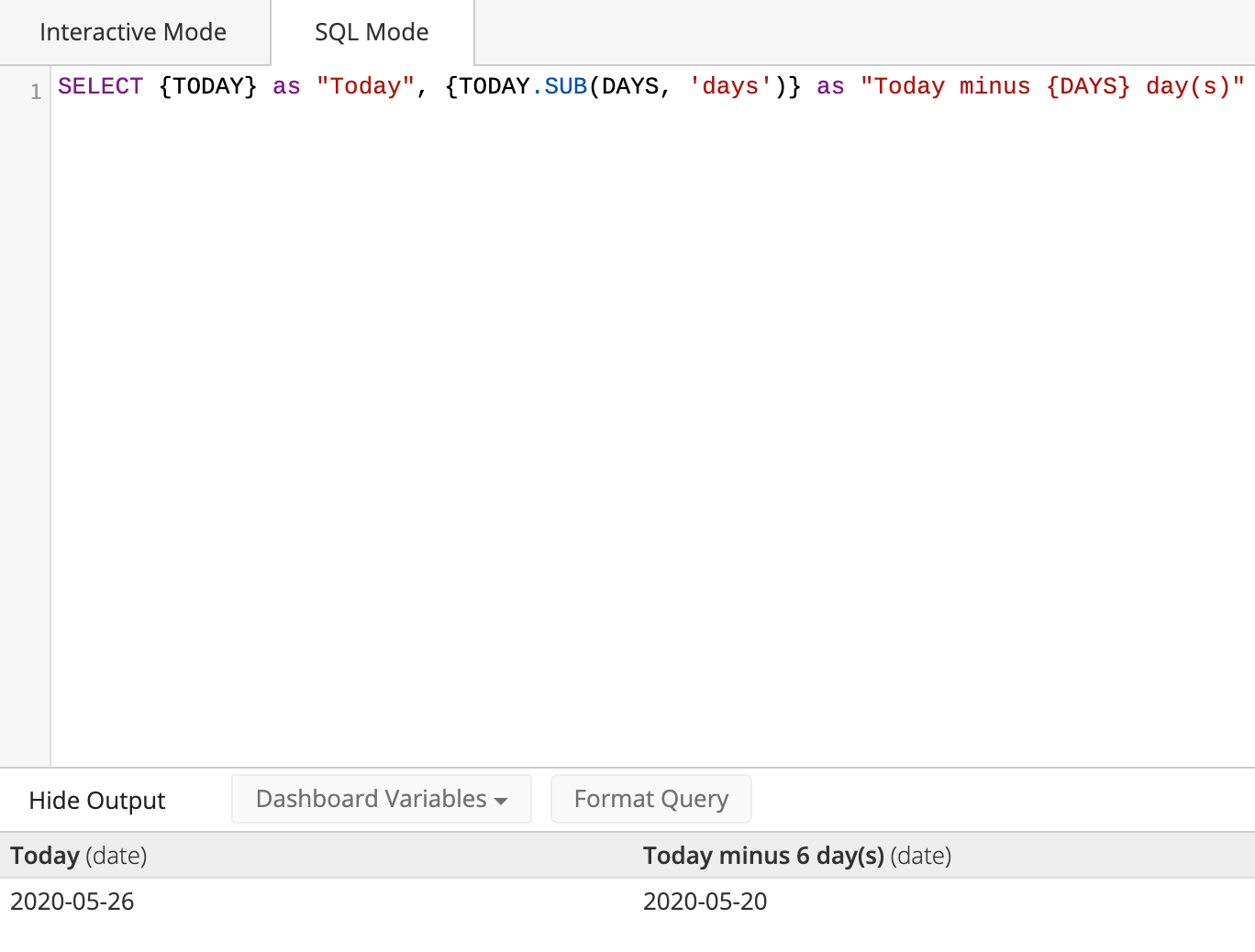# Datetime Functions

## Functions

You can perform functions on datetime Controls and variables, which allows you to customize the date returned to fit your needs.

Multiple functions can be chained together; see examples below.

To try these out in Chartio, open the Chart tab in SQL Mode and add `SELECT` before your date formula. For example:

``````SELECT {CURRENT_CALENDAR_YEAR.START.SUB(1,'day')}
``````

## Units

Units are used to perform calculations on dates (add or subtract) and extract part of a date (e.g., return only the year part of a date). They can be used in either singular or plural form and include:

• day
• week
• month
• year
• quarter (PART() only)

Add/subtract from Relative Date Variables to create other dates. Use the following format:

``````{DATE_VARIABLE.ADD(integer,'units')}
{DATE_VARIABLE.SUB(integer,'units')}
``````

For example, to get the first day of the previous month:

``````{CURRENT_MONTH.START.SUB(1,'month')}
``````

Example output:

{CURRENT_MONTH.START} {CURRENT_MONTH.START(1, ‘month’)
2020-03-01 2020-02-01

Add and subtract functions can be combined to customize dates.

## Calculating the last day of the previous month

Let’s say it’s currently June. `{CURRENT_MONTH.END.SUB(1, 'month')}` will return 2016-05-30, because we’re subtracting one month from 2016-06-30.

To get the last day of the previous month, use the following instead, which simply gets the day before the first day of the current month:

``````{CURRENT_MONTH.START.SUB(1,'day')}
``````

## Extract part of a date

We have two functions for extracting part of a date: PART() and BUCKET(). `PART()` returns a number, and `BUCKET()` returns a date string.

### PART()

`PART()` allows you to extract part of a date and returns it as a number that can be used in calculations. Use the following format:

``````{DATE_VARIABLE.PART('units')}
``````

#### PART() examples

1. Return the quarter number of the current day:

`````` {TODAY.PART('quarter')}
``````

Example output:

{TODAY} {TODAY.PART(‘quarter’)}
2020-03-30 1
2. Return the last day of the current month:

`````` {CURRENT_MONTH.END.PART('day')}
``````

Example output:

{CURRENT_MONTH.END} {CURRENT_MONTH.END.PART(‘day’)}
2020-03-31 31
3. Return the current year in number form:

`````` {TODAY.PART('year')}
``````

Example output:

{TODAY} {TODAY.PART(‘year’)}
2020-03-30 2020

### BUCKET()

`BUCKET()` allows you to extract part of a date and returns it as a date string. Use the following format:

``````{DATE_VARIABLE.BUCKET('units')}
``````

#### BUCKET() examples

1. Return the month and year of the previous month:

`````` {CURRENT_MONTH.START.SUB(1,'month').BUCKET('month')}
``````

Example output:

{CURRENT_MONTH.START} {CURRENT_MONTH.START.SUB(1,’month’).BUCKET(‘month’)}
2020-03-01 2020-02
2. Return the ISO week date of the current day:

`````` {TODAY.BUCKET('week')}
``````

Example output:

{TODAY} {TODAY.BUCKET(‘week’)}
2020-03-30 2020-W14
3. Return the quarter date of the current day:

`````` {TODAY.BUCKET('quarter')}
``````

Example output:

{TODAY} {TODAY.BUCKET(‘quarter’)}
2020-03-30 2020-Q1

## Controls nested in datetime functions

Referencing a Hidden Variable, Text Input, or some other applicable Control type within a datetime function requires you to omit the usual curly braces from the Control’s name.

For example, let’s say we have a Text Input called “DAYS” that takes in integer values and we want to use the Text Input value in a datetime function so we can dynamically select data created N days from today, where N is our Text Input value. Our function would look like this:

``````{TODAY.SUB(DAYS, 'days')}
``````
In the Visual SQL interfaceIn the Data Explorer interface